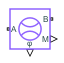# Flow Rate Sensor (MA)

Measure mass, energy, and volumetric flow rates in moist air networks

Since R2023a

•Libraries:
Simscape / Foundation Library / Moist Air / Sensors

## Description

The Flow Rate Sensor (MA) block represents an ideal sensor that measures mass flow rate, energy flow rate, and volumetric flow rate in a moist air network. There is no change in pressure, temperature, specific humidity, or trace gas mass fraction across the sensor.

Because the flow rates are Through variables, the block must connect in series with the component being measured.

The relative orientation of ports A and B establishes the measurement sign. The sign is positive if flow occurs from port A to port B. Switching port connections reverses the measurement sign.

Five physical signal ports are available to output the measurement data:

• Port M outputs the mass flow rate.

• Port V outputs the volumetric flow rate.

• Port φ outputs the energy flow rate.

• Port Mw outputs the water vapor mass flow rate.

• Port Mg outputs the trace gas mass flow rate.

These ports have conditional port visibility on the block icon. Use the block parameters to expose only the ports that you need for measurements in a particular model.

Connect the measurement ports to PS-Simulink Converter blocks to transform the output physical signals into Simulink® signals, for example, for plotting or additional data processing.

## Ports

### Output

expand all

Physical signal output port for the mixture mass flow rate measurement.

#### Dependencies

To enable this port, select the Mass flow rate check box.

Physical signal output port for the mixture volumetric flow rate measurement.

#### Dependencies

To enable this port, select the Volumetric flow rate check box.

Physical signal output port for the mixture energy flow rate measurement.

Physical signal output port for the water vapor mass flow rate measurement.

#### Dependencies

To enable this port, select the Water vapor mass flow rate check box.

Physical signal output port for the trace gas mass flow rate measurement.

#### Dependencies

To enable this port, select the Trace gas mass flow rate check box.

### Conserving

expand all

Moist air conserving port. Flow rates are positive if air flows from port A to port B.

Moist air conserving port. Flow rates are positive if air flows from port A to port B.

## Parameters

expand all

Selecting this check box exposes the output port M, which lets you measure mass flow rate.

Selecting this check box exposes the output port V, which lets you measure volumetric flow rate.

Select whether the sensor calculates volumetric flow rate at actual working conditions or at a standard pressure, temperature, and relative humidity:

• `Actual conditions` — The sensor calculates the volumetric flow rate by using the moist air density at the actual working conditions of the system.

• `Standard conditions` — The sensor calculates the volumetric flow rate by using the moist air density at standard conditions. When you select this option, additional parameters become available to let you specify the standard pressure, temperature, and relative humidity.

#### Dependencies

To enable this parameter, select the Volumetric flow rate check box.

Moist air pressure at standard conditions.

#### Dependencies

To enable this parameter, set Volumetric flow measurement conditions to `Standard conditions`.

Moist air temperature at standard conditions.

#### Dependencies

To enable this parameter, set Volumetric flow measurement conditions to `Standard conditions`.

Moist air relative humidity at standard conditions.

#### Dependencies

To enable this parameter, set Volumetric flow measurement conditions to `Standard conditions`.

Selecting this check box exposes the output port φ, which lets you measure energy flow rate.

Selecting this check box exposes the output port Mw, which lets you measure water vapor mass flow rate.

Selecting this check box exposes the output port Mg, which lets you measure trace gas mass flow rate.

## Version History

Introduced in R2023a

expand all